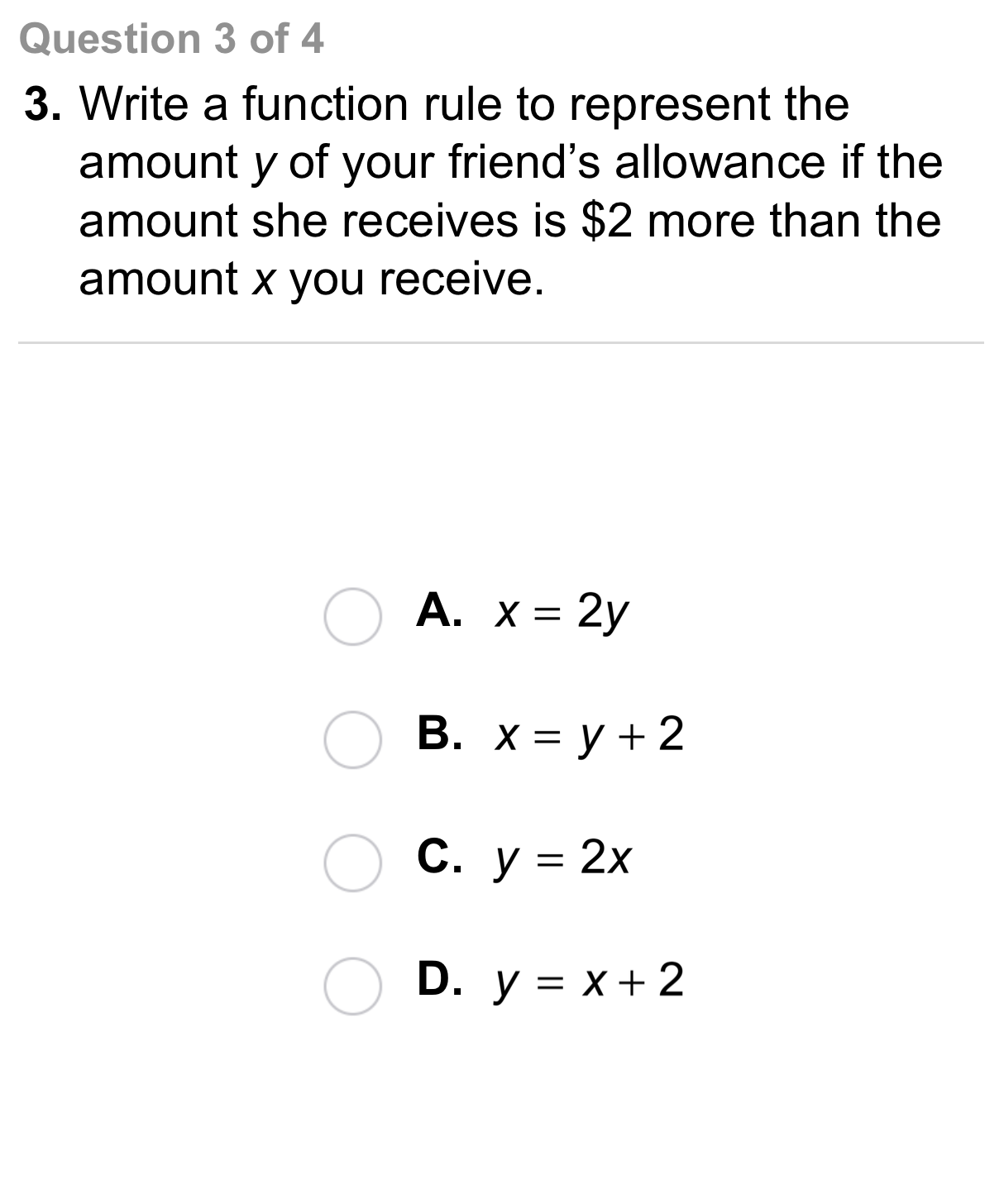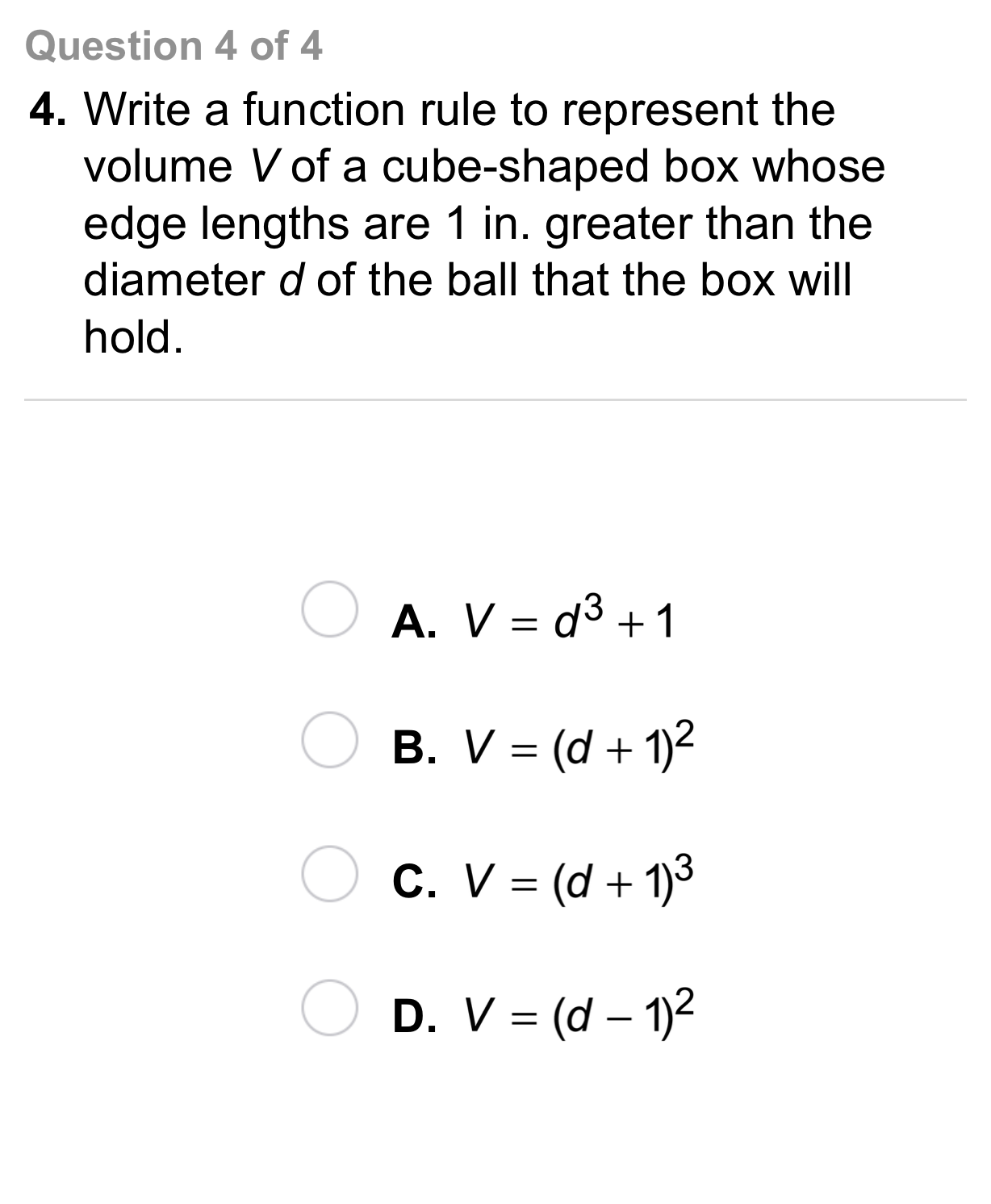Algebra 1 4-5 Independent Practice: Writing a Function Rule
starstarstarstarstarstarstarstarstarstar
by Matthew Richardson
| 9 Questions1
1
10
A
B
C
D2
2
10
A
B
C
D3
3
10
A
B
C
D4
4
10
A
B
C
D5
5
Vocabulary: Suppose you write an equation that gives a as a function of b. Which is the dependent variable?
a
b
6
10
Error Analysis: A worker has dug 3 holes for fence posts. It will take 15 min to dig each additional hole. Your friend writes the rule t = 15n + 3 for the time t, in minutes, required to dig n additional holes. Describe and correct your friend's error.
7
5
Reasoning: Is the graph of a function rule that relates a square's area to its side length continuous or discrete?
continuous
discrete
9
10
Reflection: Math Success